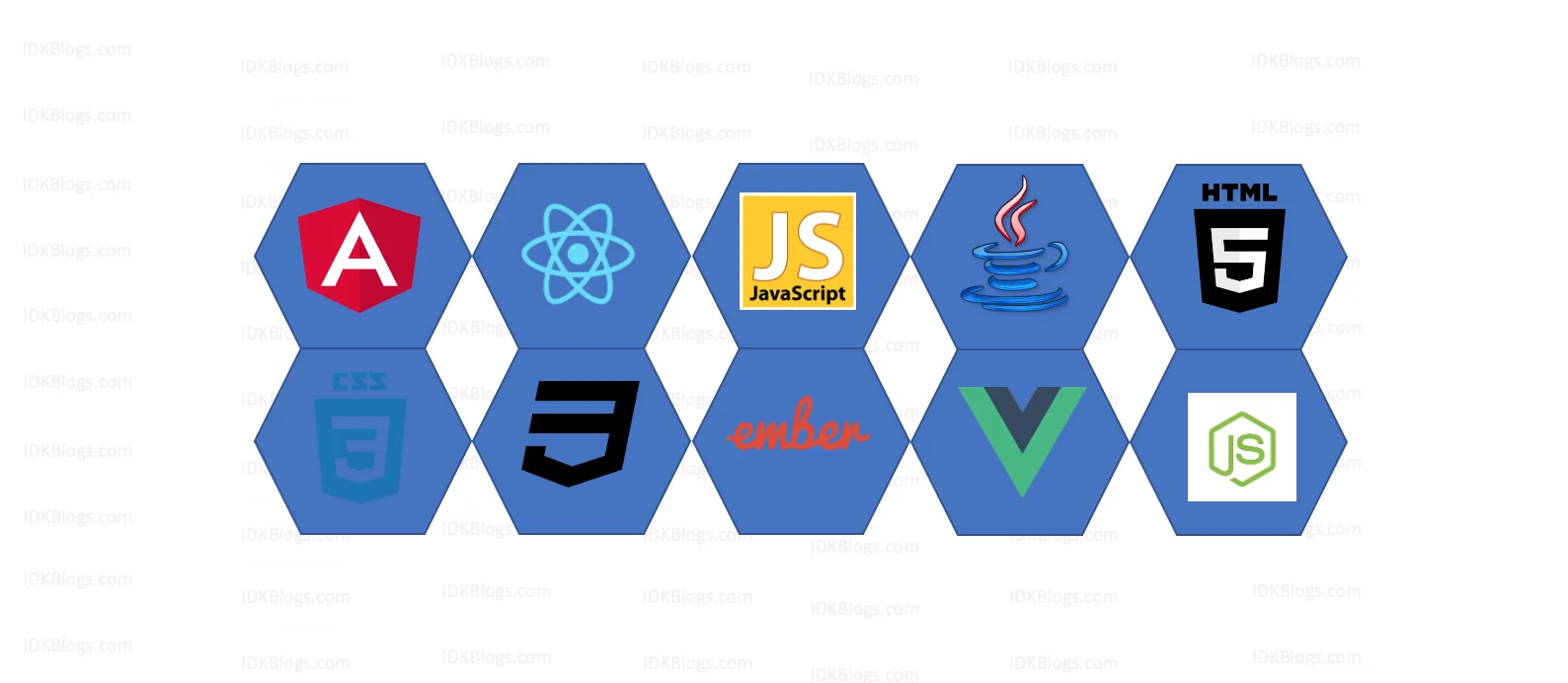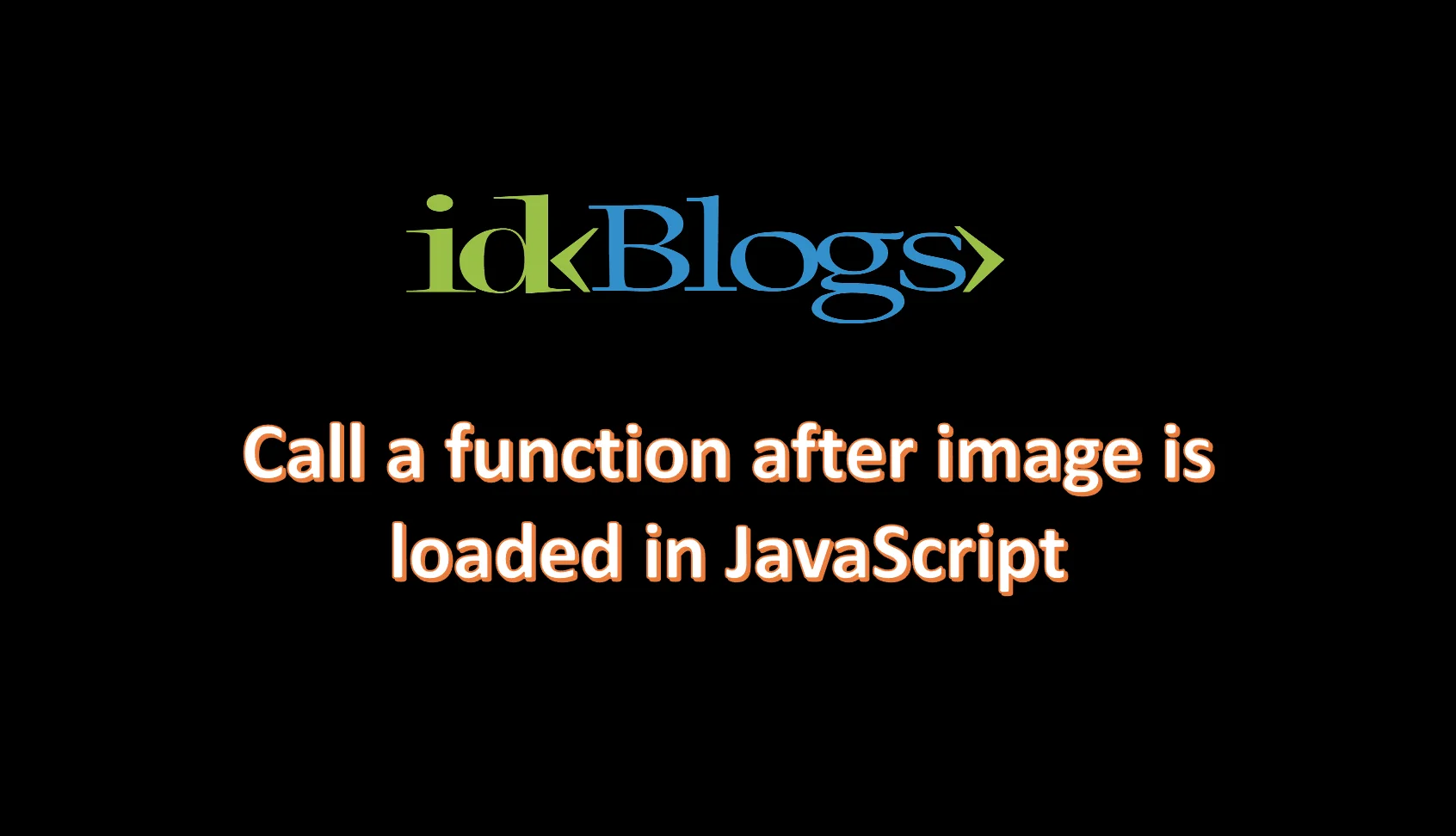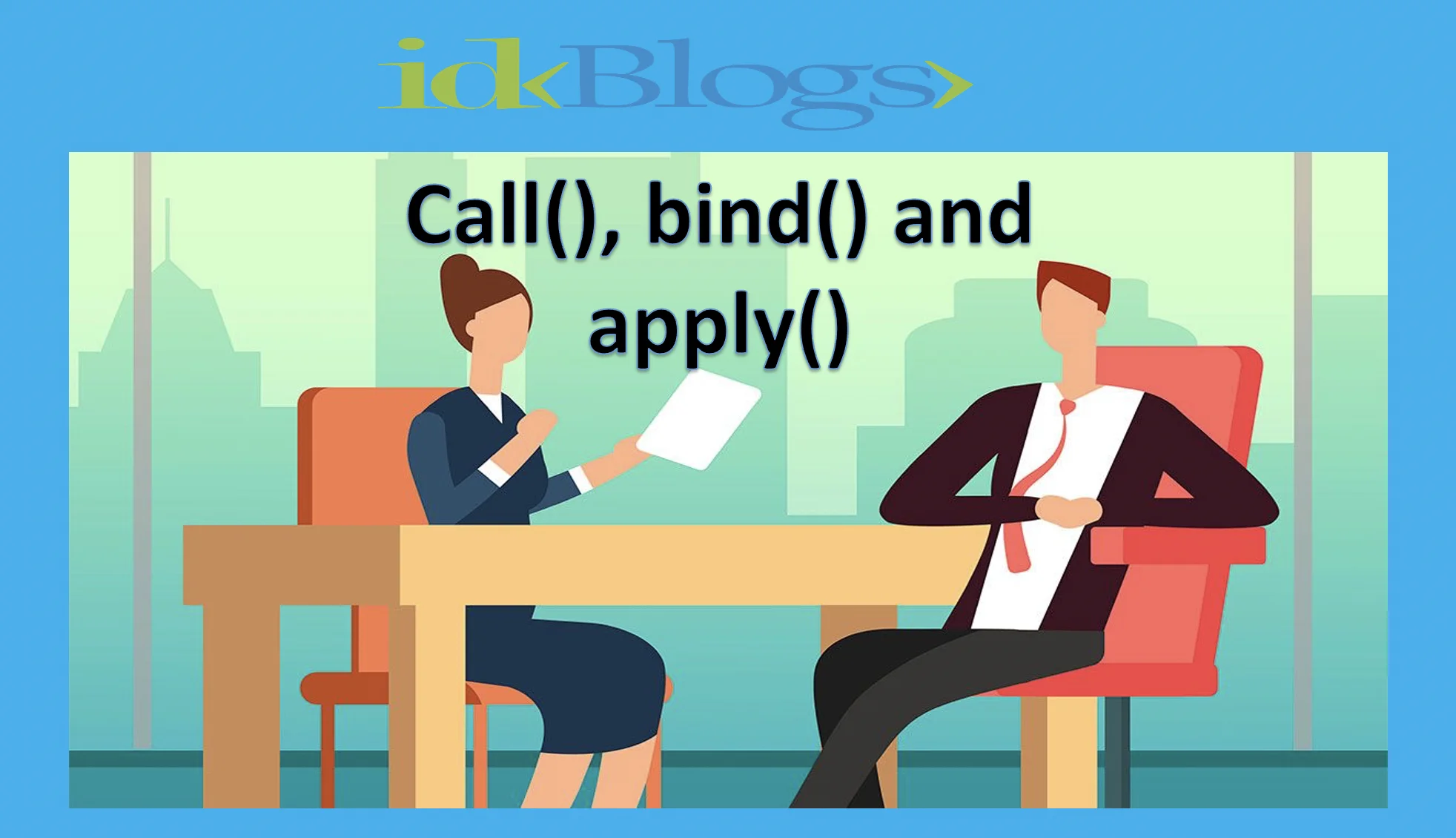# Closures in JavaScript

### What are the Closures in JavaScript? When to use Closures? Concepts of Closures in JavaScript

idkblogs.com      August 27, 2021Shubham Verma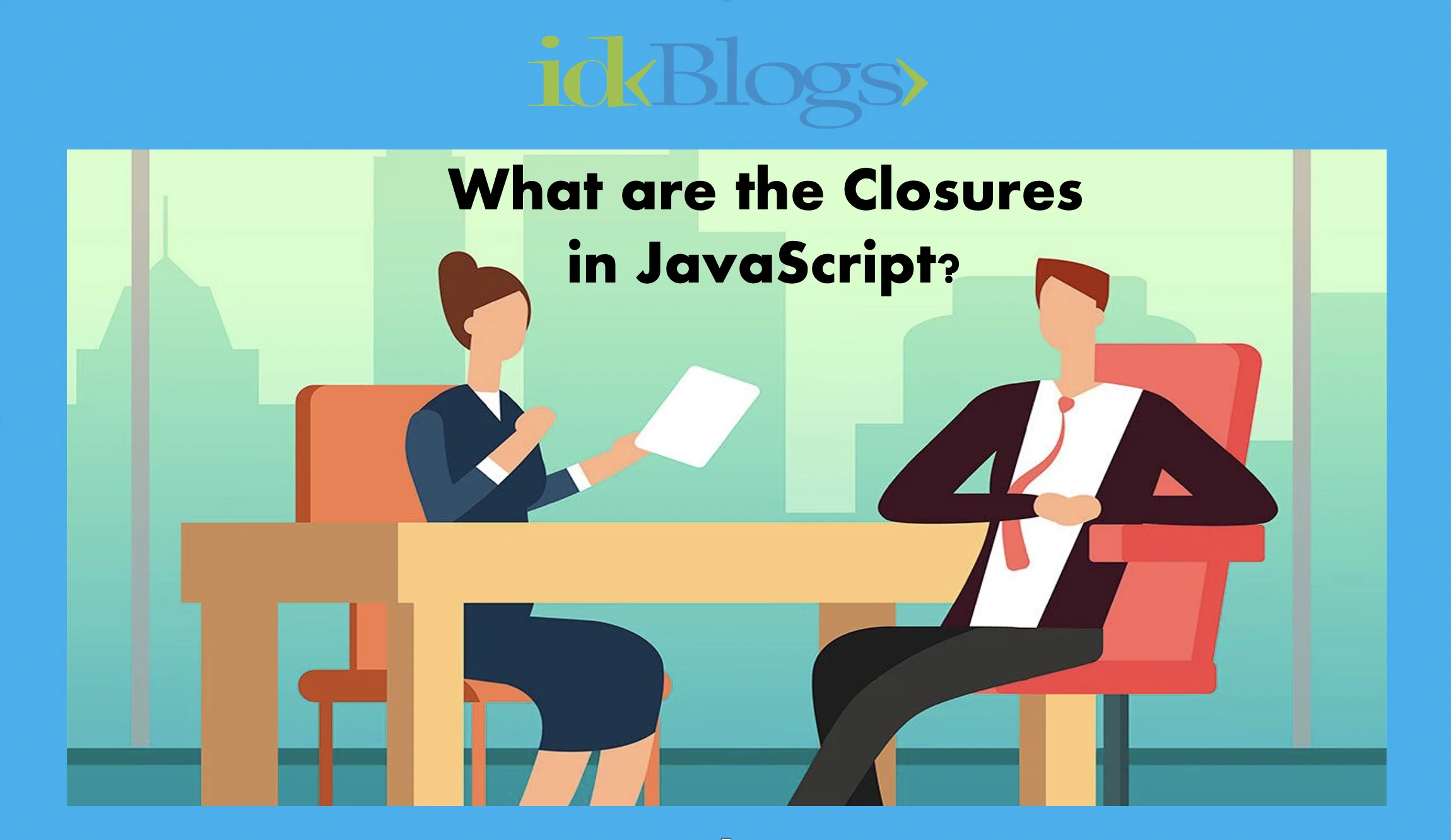# What are the Closures in JavaScript?

In this tutorial, you'll have a good knowledge about the Closures and you won't have to go to any other tutorial to get about the Closures. Closures basically a function bind together with its Lexical Environment (function along with the lexical scope).

Let's get started and have a look into the below codes:
``````                      function x(){
var a =7;
function y(){
console.log(a);
}
y();
}

x();
``````

The function "y" binds to the variables of x, So that means in the above code, the function "y" forms a closure and the function "y" has access to its parent's lexical scope. Let's run the above code and see in the browser: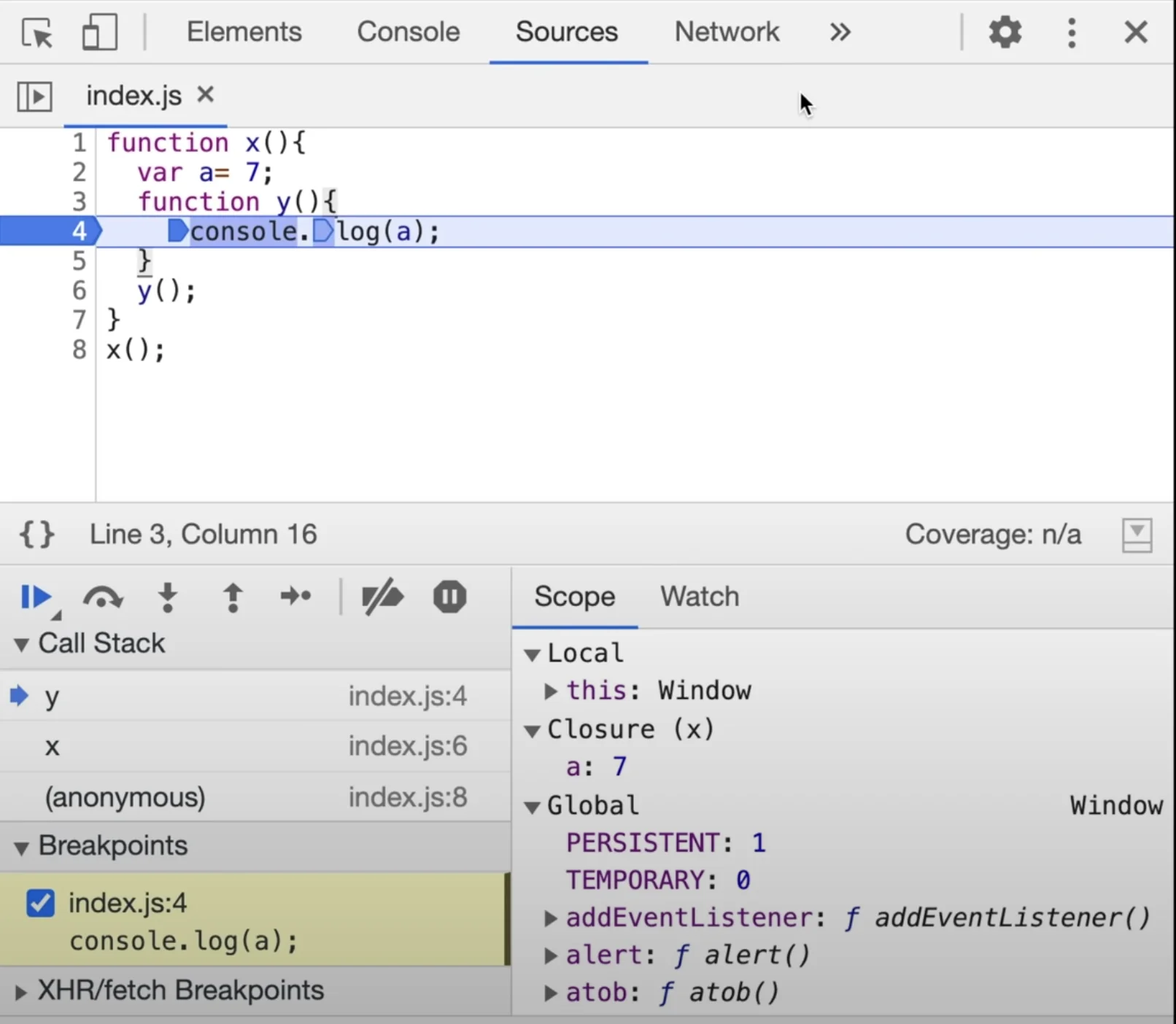# What are the Closures in JavaScript?

You can see in the above image, the scope of the function "y" has closure and in that closure, it has a variable "a" with value 7. And also it has a global reference which is the reference of its parent's lexical environment.

In JavaScript, you can do many things with function, like can pass as an argument, can store in a variable, can return, etc. So when you return a function in a function (nested function), then closure gets more complicated. So, let's write the code where we'll return a function:
``````                      function x(){
var a =7;
return function(){
console.log(a);
}
}

const z = x();
z();
``````

In the above codes, in the variable z, the returned function will be stored outside the scope. Now we are calling z. What will happen with z();

The z() will try to access the 'a', but a is not in the global scope and x is no longer exists. So will happen to this 'a'?

What will be the output? What it will print ?

It will print 7;

WoowWWW..

So here comes closure into the picture, When the function is returned from other functions they still maintain their lexical scope. They remember where they actually were present. So in the above code, the function 'y' will remember the lexical environment. And that's why the function 'y' will remain access the variable 'a' and its value '7'.

In shorts, when a function was returned, it's not just the codes that were returned, basically, it returns the closure and the closure enclosed that function and its lexical scope. And here we have stored in the variable 'z', and when we execute 'z', it still remembers the reference to variable 'a' and try to access the value of 'a'. So this is the closure, function along with it lexical environment bundled together forms a closure.

## 1.

``````                      function x(){
var a =7;
function y(){
console.log(a);
}
a=100;
return y;
}

const z = x();
z();
``````

What will be the output:
100

Because variable 'a' doesn't refer to the value, it's a reference to variable 'a'. So when you want to access the variable 'a' it will see what is the current value on that memory location and will get those values.

## 2.

``````
function z(){
var b=900;
function x(){
var a =7;
function y(){
console.log(a,b);
}
y();
}
x();
}

z();

``````

In the above code, what will be the output of the console?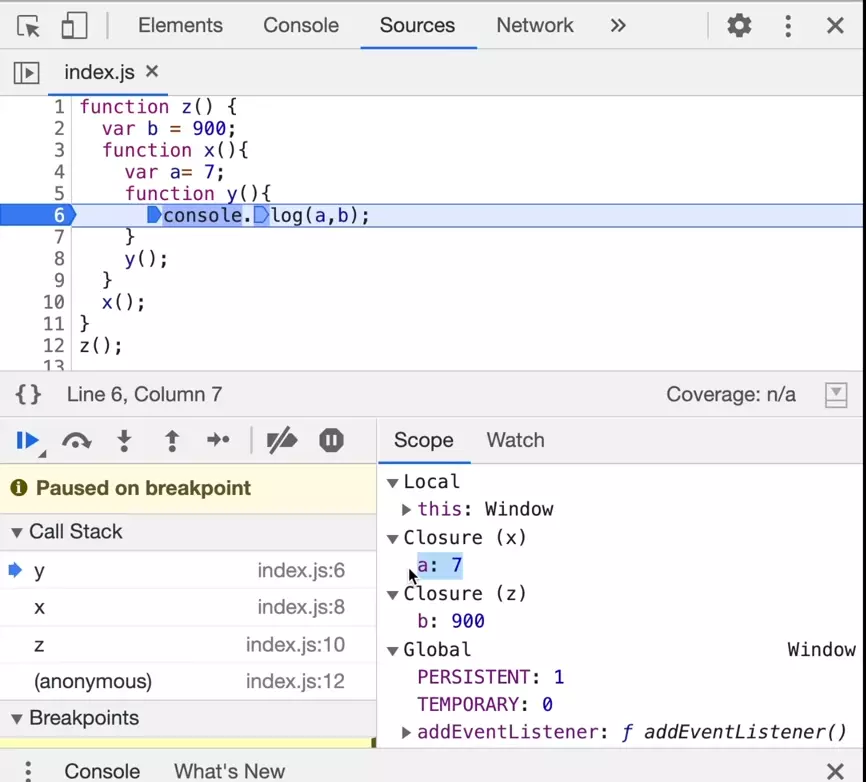# What are the Closures in JavaScript?

Output: 7 900
It means, y() forms a clouser along with scope of x() and scope of z().

## Uses of closures: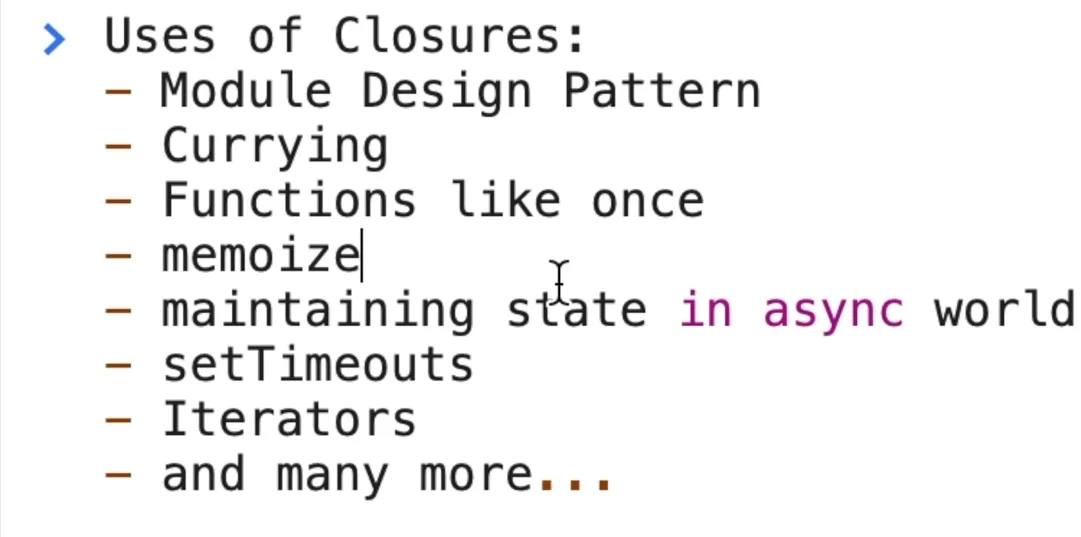# What are the Closures in JavaScript?

### Conclusion:

In this tutorial, we learned about closures and their uses. Also, we have seen how clouser works.

Thank you

To read more about the technologies, Please subscribe us, You'll get the monthly newsletter having all the published article of the last month.

### OTHER POSTS